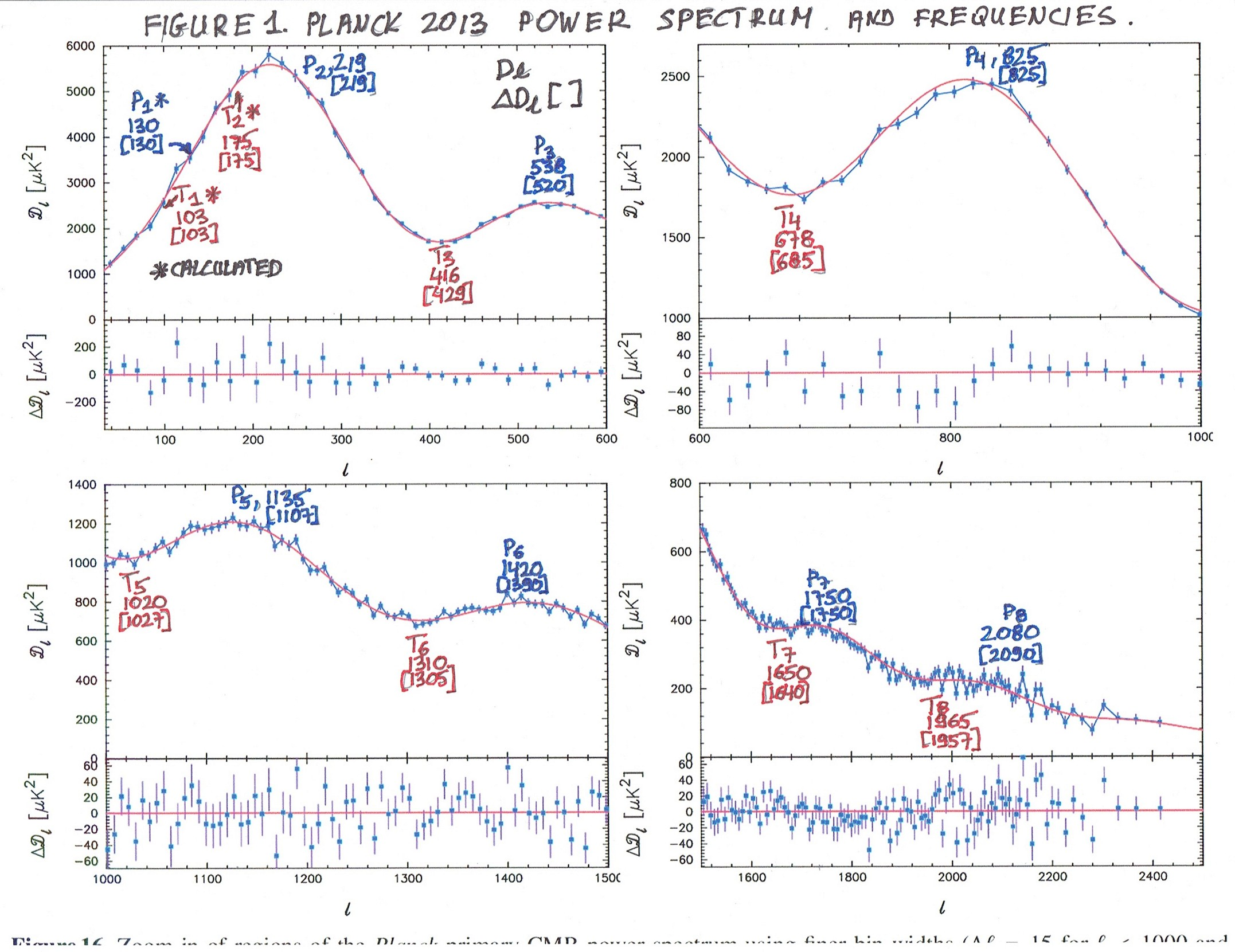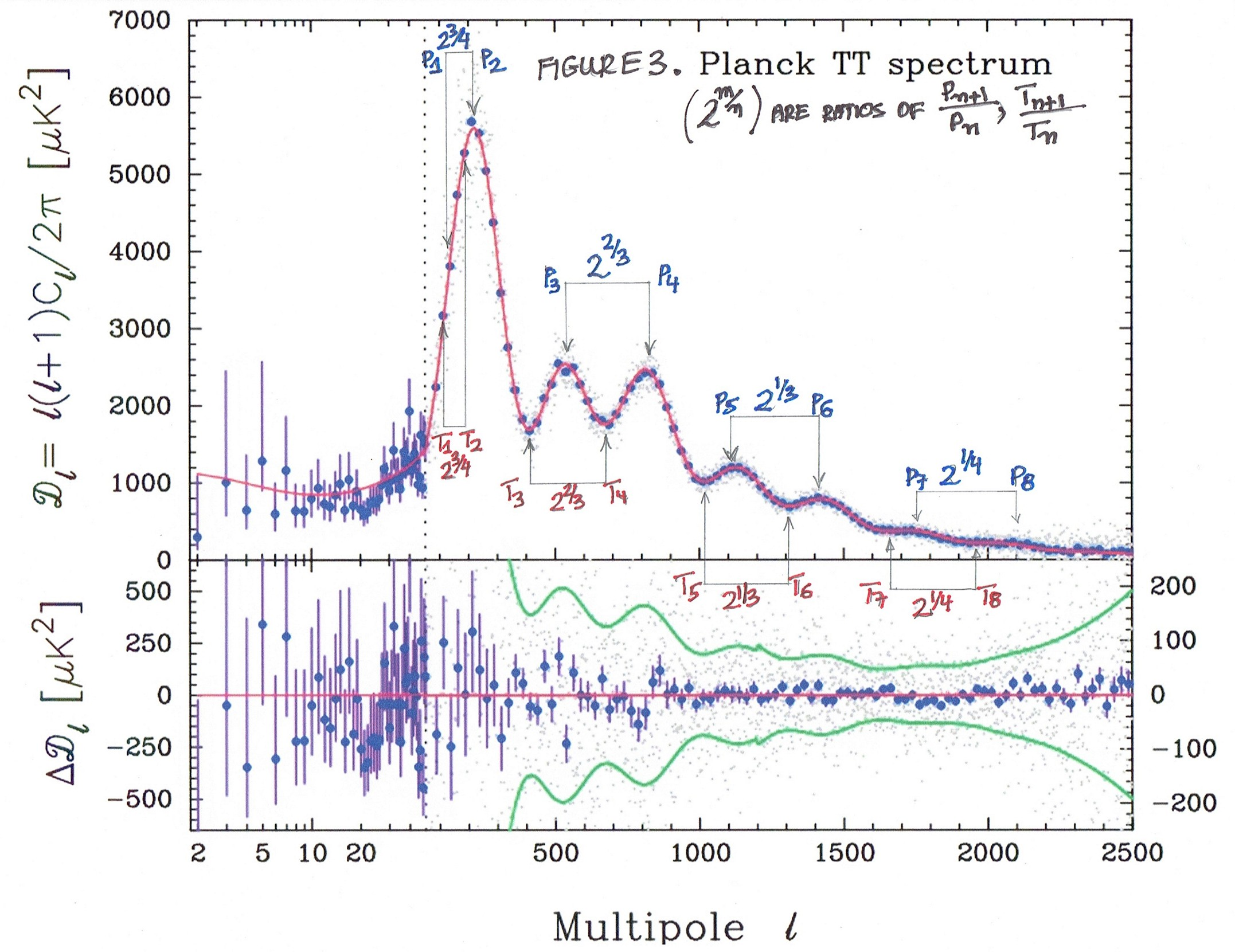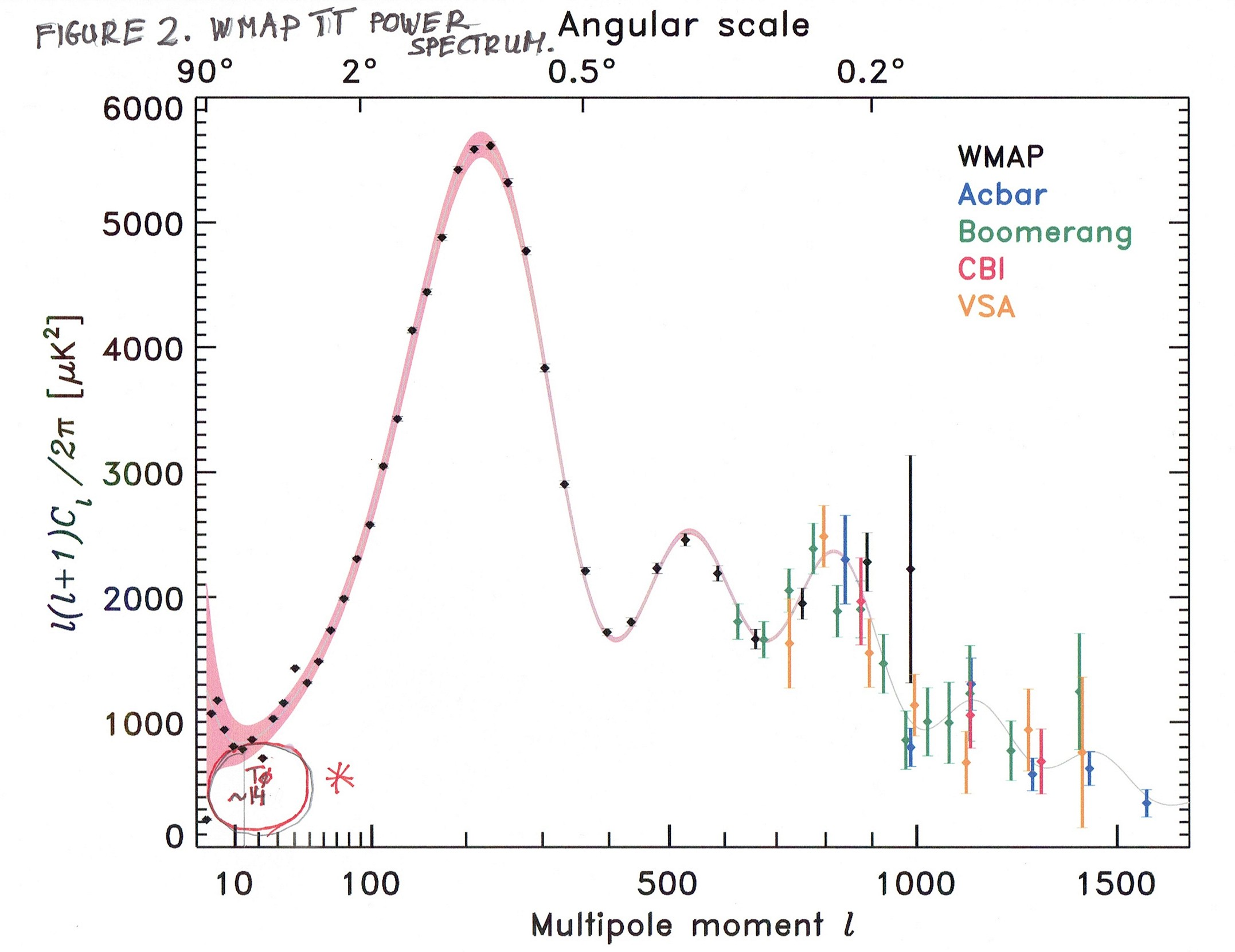15 June 2020 AD;

St Vitus, St Modestus and St Crescentia (c. 300)

St Germaine Cousin (1601)

In this article, I will derive the first sounds in the Universe based on the Cosmic Microwave Background (CMB) which is so to speak the earliest picture of our Universe.

From Figure 1. we can see that frequencies in Hertz might be assigned to the Peaks and Troughs (see the article Creation of the Universe and Music. Part I  ).

Here is again Figure 1. Planck 2013 Power Spectrum and Frequencies.We can see the ratios of the frequencies for Peaks and Troughs. (Numbers in square brackets are from the lower portion of the Graph.)

Peaks:

P8 / P7 = 2080 / 1750 = 21/4

P6 / P5 = 1420 / 1135 = 20.323 and  /  = 20.328 which is approximately equal to 21/3

P4 / P3 = 825 / 538 = 20.617 and  /  = 22/3

The next ratio should be 23/4 . I will come back to it later.

Troughs:

T8 / T7 = 1965 / 1650 = 21/4 and  /  = 20.255

T6 / T5 = 1310 / 1020 = 20.361 and  /  = 20.347 which is approximately 21/3

T4 / T3 = 678 / 416 = 20.705 and  /  = 20.675 which is approximately 22/3

The next ratio should be also as in the previous case equal to 23/4

This ratios and frequencies can be seen clearly on the next picture:

Figure 3. Planck TT Power Spectrum.Now, there is a simple relation between those ratios. If you consider every second ratio, we get this result:

(22/3 ) / (21/4 ) = 28/12 - 3/12 = 25/12

and starting with the ratio 21/3 :

( 21/3 ) X ( 25/12 ) = 24/12 + 5/12  = 29/12 = 23/4

So the multiplication/division factor between every second ratio equals +/- 25/12

Now we can get frequency P1 :

P2 / P1 = 219 / x .

From this we have P1 = 130.22

This factor 25/12 suggests immediately a couple of things:

1 - the denominator of the fraction 5/12 is 12 so it suggests that we have 12 notes in an octave (similar to piano)

2 - Each next tone will be different from the previous one by a factor +/- 21/12

The Tests for frequencies:

1 - To get all the frequencies with some good approximation (which requires some luck as well), we will have to get for each Peak and Trough frequency the same series of musical notes. This way we will be able to calculate the Fundamental Frequency.

2 - Another test will be as follows: From Figure 2. (below) we can clearly see that there is a Trough at the value 13-14 at the beginning of the X-axis. The test is: all the calculations from Peaks and Troughs should give us this value (greater than 13, and less than 14).

Figure 2. WMAP TT Power Spectrum:We can see immediately that frequency of P1 = approximately 130 Hz, gives the following multiplications:

2X130 = 260

2X260 = 520 (frequency P3 )

2X520 = 1040 (frequency P6 )

2X1040 = 2080 (frequency P8 )

These results suggest strongly the Fundamental Frequency in the middle of the piano C4 = approx. 260 Hertz.

Having the FF (Fundamental Frequency) we can calculate the value of 13-14 Hertz from Figure 2. It should be a fractional power of 2

260 / (251/12 ) = 13.6646 Looks O.K.

To be sure, one step below and above:

260 / (250/12 ) = 14.48 (too much)

260 / (252/12 ) = 12.90 (not enough)

Now we can get with good approximation the values of all frequencies of the Peaks and Troughs. Here is the list of the frequencies:

P8 = 260 X 236/12 = 2080 (approx. C7 on the piano)

check:

P8 / PF = 2080 / 13.66457 = 287/12 Good result.

P7 = 260 X 233/12 = 1749.07 Hz (approx. A6 on piano)

P6 = 260 X 229/12  = 1388.23 Hz (approx. F6 on the piano)

P5 = 260 X 225/12 = 1101.84 Hz (approx. C sharp 6 on the piano)

P4 = 260 X 221/12 = 874.53 Hz (approx. G sharp 5 on piano)

P3 = 260 X 212/12 = 520.00 (approx. C5 on piano)

P2 = 260 X 2-3/12 = 218.63 Hz (approx. A3 on piano)

P1 = 260 X 2-12/12 = 130.00 Hz (approx. C3 on piano)

This procedure gives us 8 sounds (Peaks)

Now the sounds from the Troughs:

T8 = 260 X 235/12 = 1963.26 Hz (approx. B6 on piano)

T7 = 260 X 232/12 = 1650.90 Hz (approx. G sharp 6)

T= 260 X 228/12 = 1310.32 Hz (approx. E6 on piano)

T5 = 260 X 224/12 = 1040.00 (approx. C6 on the piano)

T4 260 X 217/12  = 694.12 Hz (approx. F5 on the piano)

I calculated ("educated guess") some of the missing frequencies and used exact values of the first frequencies. If you want to listen to these 20 sounds here is the YouTube video:

The First Sounds Ever from CMB

If you prefer to play approximate music notes on piano, you can do it here:

Virtual Piano

In the next article, I will show how to get the exact frequencies of the whole music scale, which is coded into these 13 sounds. And after that more stuff coming up.